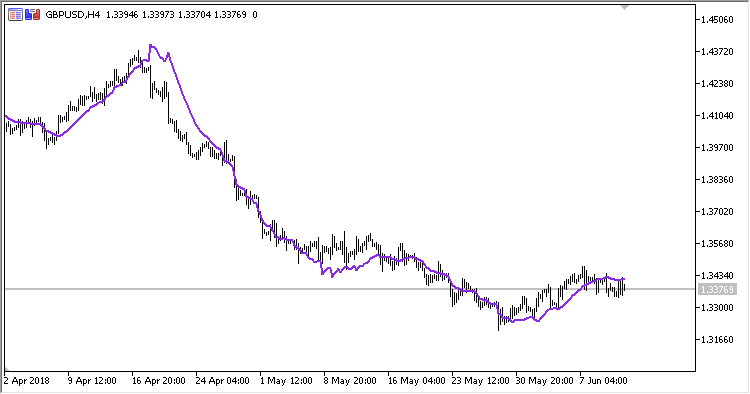• 加入 >>291

2018.07.13 10:44

• RSI period - RSI 周期.
• MA period - 均线方法;
• Method - 计算方法;
• Applied price - 计算所用价格类型。

ReRSI = Applied price + X * (100.0 - RSI_MA) / RSI_MA)

ReRSI = Applied price + X

X = (RSI Period - 1.0) * (ADC_MA * RSI_MA / (100.0 - RSI_MA) - AUC_MA)

X = 0
```RSI_MA = MA(RSI, MA Period, Method)
RSI = RSI(RSI Period)
AUC_MA = EMA(UP, 2*RSI Period-1)
```

UP = Applied price - PrevPrice

DN = PrevPrice - Applied priceVAMA_MACDVAMAPGCExp_UltraAbsolutelyNoLagLwma